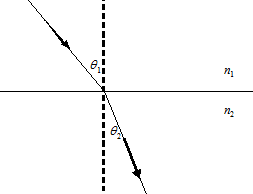### Error analysis and math

#### Problem:

An experimentalist makes independent measurements of the length and height of a rectangular feature.  The values and their standard deviations are 10.62 ± 0.46 microns and 12.46 ± 0.52 microns.  Calculate the perimeter P and the area A of the feature including the standard deviation of each.

Solution:

• Concepts:
Propagation of errors
• Reasoning:
We are asked to propagate experimental errors.
• Details of the calculation:
P = 2 * (10.62 + 12.46) ± 2 * (0.462 +0.522) ½
= 46.16 ± 1.39 microns
(In addition and subtraction, the sum or the difference cannot be stated to more places after the decimal than the term with the least number of places after the decimal.)
A = 10.62 * 12.46 ± ((0.46/10.62)2 + (0.52/12.46)2) ½ * 10.62 * 12.46
= 132.33 ± 7.96
= 132.3 ± 8.0 square microns
(When numbers are multiplied or divided, the number of significant figures in the product or quotient cannot exceed that of the least precise number used in the calculation.)
More rules:
When using logarithms, the significant figures are those in the mantissa (the figures after the decimal); the characteristic (figure before the decimal) indicates the placement of the decimal in the original number: e.g. log(2731) = 3.4363.

#### Problem:

Propagation of Errors:  Snell's law relates the angle of refraction θ2 of a light ray travelling in a medium of index of refraction n2 to the angle of incidence θ1 of a ray travelling in a medium of index n1, as shown in the figure.From the knowledge that n1 = 1.000 and from independent experimental measurements of
θ1 = (22.03 ± 0.8)o, and θ2 = (10.45 ± 0.8)o, find
(a) n2 ;
(b) the percentage errors in sinθ1 and in sinθ2;
(c) the uncertainty in n2.

Solution:

• Concepts:
Propagation of errors
• Reasoning:
We are asked to propagate experimental errors.
• Details of the calculation:
(a)  sinθ1 = n2sinθ2,  n2 = sinθ1/sinθ2 = 2.07
(b) d(sinθ)/sinθ = cotθdθ
percentage error sinθ1:  3.5%
percentage error sinθ2:  7.6%
(c)  Δn2 = n2*((Δ(sinθ1)/sinθ1)2 + (Δ(sinθ2)/sinθ2)2)½ = 0.17

#### Problem:

A force F is applied on a square plate of side L.  If the percentage error (standard deviation) in determination of L is 3% and that in F is 4%, what is the error in pressure?

Solution:

• Concepts:
Error propagation for uncorrelated variables
• Reasoning:
We are asked to propagate the error in a set of measurements.
• Details of the calculation:
Pressure P = F/A = F/L2.
(∆P/P)2 = (∆F/F)2 + (∆L2/L2)2 = (∆F/F)2 + (2∆L/L)2 = 0.042 + 0.032 = 0.0025
∆P/P = 0.05.  The error in the pressure is 5%.

#### Problem:

Suppose C is the capture rate of dark matter in an astrophysical body.  Let CA be the dark matter annihilation rate per effective volume.  Then an approximate Boltzmann equation governing the number N of dark matter particles in the astrophysical body is
dN/dt = C - CAN2.
If N(0) = 0, find N(t).
Hint:  ∫du/(1 - u2) = tanh-1u

Solution:

• Concepts:
Solving a differential equation
• Reasoning:
The rate equation is given.
• Details of the calculation:
dN/(C - CAN2) = dt.  ∫0N dN'/(C - CAN'2) = ∫0t dt' = t.  Let y = (CA/C)½N.
(CCA)0y du/(1 - u2) = (CCA) tanh-1((CA/C)½N) = t
(C/CA)½tanh((CAC)½t) = N(t)
When t >> (CCA) then N(t) --> (C/CA)½